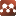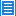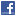Classical PhysicsThe Physical Nature of Potential Energy

Authors: Guido F. Nelissen

‘Potential’ energy is defined as the energy that has to do with location relative to something else and therefore it is also called ‘energy of configuration'. The present handbooks don’t however tell us anything about the physical nature of this so-called ‘potential’ energy and how and where it is physically stored. The only thing that we know is that in the special case of conservative forces, the disappeared kinetic energy that is conserved as ‘potential’ energy, can be completely retransformed into kinetic energy. In his paper part 3, the author has demonstrated that the kinetic energy of a particle system is a mathematical expression of its total amount of reversibly transferable translational motion. In this paper, the author demonstrates that the present concepts of kinetic and potential energies are not different kinds of energy, but that it are just different mathematical equations for the work done by a force on a given mass over a given distance: the first expressed in function of the obtained speed, and the second in function of the covered distance. This allows him to demonstrate that the present concept of gravitational ‘potential’ energy is an excellent mathematical tool to calculate the speed of falling objects in function of the covered distance, but that it creates a physical problem with the law of conservation of energy, because it supposes that both ‘kinetic’ and ‘potential’ energies arise out of nothing while the total amount of energy remains invariably zero! This leads the author to demonstrate that the reversible transformation of ‘kinetic’ energy into ‘potential’ energy and vice versa, is in fact a mathematical expression for the reversible transformation of one kind of congruent motion into another, which allows him to demonstrate that the ‘potential’ energy of a perfectly elastic spring, is a mathematical expression of the amount of coherent, internal rotational-vibrational motion of its basic mass particles.

Submission history

[v1] 2019-04-25 14:00:00
[v2] 2019-09-19 15:07:49